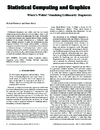Michael Friendly's

# Where's Waldo: Visualizing Collinearity Diagnostics• Where's Waldo: Visualizing Collinearity Diagnostics.
• The American Statistician, vol. 63, no. 1, pp. 56–65, 2009.

Collinearity diagnostics are widely used, but the typical tabular output used in almost all software makes it hard to tell what to look for and how to understand the results. We describe a simple improvement to the standard tabular display, a graphic rendition of the salient information as a ``tableplot,'' and graphic displays designed to make the information in these diagnostic methods more readily understandable. In addition, we propose a visualization of the contributions of the predictors to collinearity through a \emphcollinearity biplot, which is simultaneously a biplot of the smallest dimensions of the correlation matrix of the predictors, \matR_XX, and the largest dimensions of \matR_XX^-1, on which the standard collinearity diagnostics are based.

Keywords: condition indices; collinearity biplot, diagnostic plots, effect ordering, multiple regression, tableplot, variance inflation

```@Article{FriendlyKwan09collin,
author = {Michael Friendly and Ernest Kwan},
title = {Where's Waldo: Visualizing Collinearity Diagnostics},
journal = {The American Statistician},
year = {2009},
volume = {63},
number = {1},
pages = {56–65},
doi = {10.1198/tast.2009.0012},
url = {http://datavis.ca/papers/viscollin-tast.pdf},
supp = {http://datavis.ca/papers/viscollin/},
}```